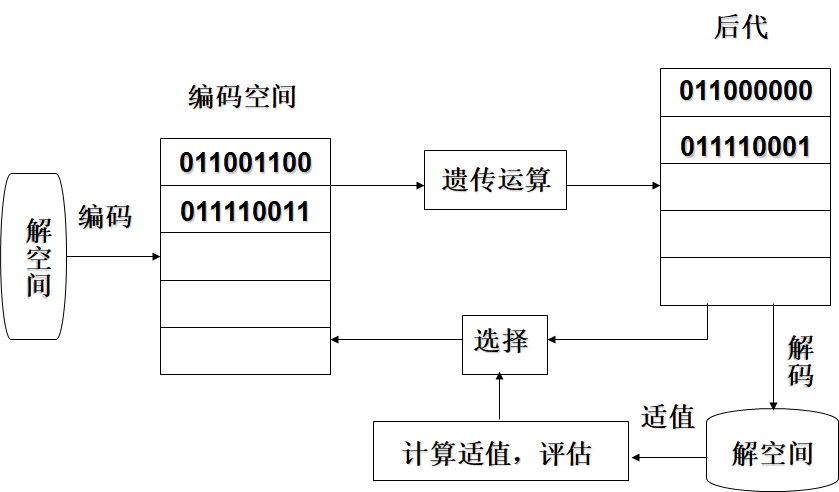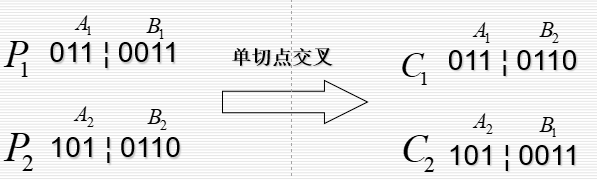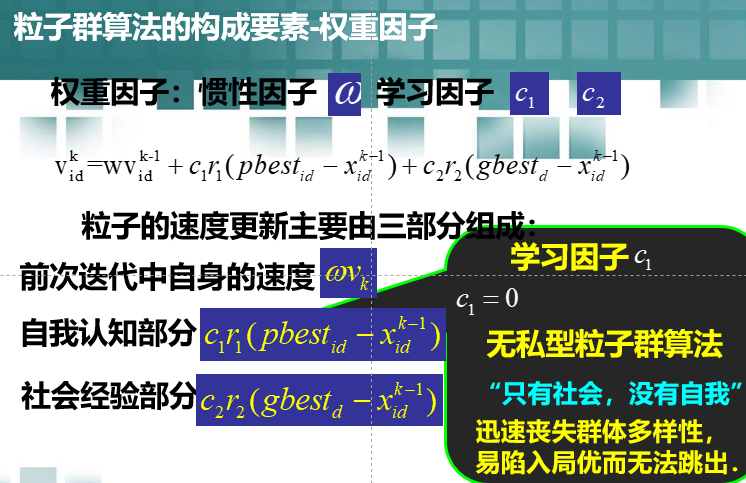# 智能优化算法

## 遗传算法

Genetic Algorithm，简称GA

• 基本思想：

• 根据问题的目标函数构造适值函数Fitness Function
• 产生一个初始种群
• 根据适值函数的好坏，不断的进行选择繁殖
• 若干代后得到适值函数最好的个体即为最优解。
• 构成要素：

• 种群 population 种群大小 pop-size

• 种群表达法 – 编码方法

• 遗传算子 genetic operator

交叉 crossover 变异 mutation

交叉率高，解空间大，但计算时间较长

• 选择策略

一般为正比选择

选择种群中适值高的个体，适者生存

• 停止准则

一般是指定最大迭代次数

• 解空间与编码空间的转换• 初始种群的产生
• 编码方法
• 适值函数
• 遗传算法
• 选择策略
• 停止准则

$\Delta$ 初始种群的产生

​ 产生$\zeta_i\in U(0,1)$

​ $\zeta_i>0.5,\quad x_i=1;$

​ $\zeta_i<0.5,\quad x_i=0;$

$\Delta$ 编码方法 –二进制编码

$\Delta$ 适值函数–根据目标函数设计

$\Delta$ 遗传运算–选择、交叉、变异

$\bigstar$ 交叉 Crossover

​ $\heartsuit$ 单切点交叉

​ 随机产生一个断点 $[1,n-1]$​ $\heartsuit$ 双切点交叉

$\bigstar$ 变异 Mutation

​ 初始种群中没有需要的基因，在种群中按变异概率$\ P_m$任选若干位基因改变位值0→1或1→0，

$\bigstar$ 选择

​ 最常用的正比选择

​ 对于个体$i$，适值$F_i$，选择概率如下公式计算
$$P_i={F_i \over{\sum_{1}^{NP}F_i}}$$

$$NP–Number of Population$$

​ 之后采用轮盘赌的方法进行选择：

​ 令$PP_0=0，PP_i=\sum_{j=1}^{i} P_j$

​ 随机产生 $\varepsilon_i \in U(0,1)$

​ 当 $PP_i \le \varepsilon_i \le PP_i$,选择个体 $i$，

## 粒子群算法

Particle Swarm Optimization

• 基本思想

• 粒子群算法q粒子群算法的思想源于对鸟群捕食行为的研究

• 模拟鸟集群飞行觅食的行为，鸟之间通过集体的协作使群体达到最优目的，是一种基于Swarm Intelligence的优化方法。

• 马良教授在他的著作《蚁群优化算法》一书的前言中写到：

“自然界的蚁群、鸟群、鱼群、羊群、牛群、蜂群等，其实时时刻刻都在给予我们以某种启示，只不过我们常常忽略了大自然对我们的最大恩赐！……”

• 算法介绍

• 每个寻优的问题解都被想像成一只鸟，称为“粒子”。所有粒子都在一个D维空间进行搜索。
• 所有的粒子都由一个fitness function 确定适应值以判断目前的位置好坏。
• 每一个粒子必须赋予记忆功能，能记住所搜寻到的最佳位置。
• 每一个粒子还有一个速度以决定飞行的距离和方向。这个速度根据它本身的飞行经验以及同伴的飞行经验进行动态调整。

D维空间中，有N个粒子；

​ 粒子$i$位置：$x_i=(x_{i1},x_{i2},\cdots x_{iD})$，将$x_{i}$代入适应函数$f(x_i)$求适应值；

​ 粒子$i$速度：$v_i=(v_{i1},v_{i2},\cdots v_{iD})$

​ 粒子$i$个体经历过的最好位置：$pbest_i=(p_{i1},p_{i2},…p_{iD})$

​ 种群所经历过的最好位置：$gbest=(g_1,g_2,…g_D)$

• 粒子$i$的第$d$维速度更新公式：
$$v_{id}^{k}=\omega v_{id}^{k-1}+c_1r_1(pbest_{id}-x_{id}^{k-1})+c_2r_2(gbest_d-x_{id}^{k-1})$$

• 粒子$i$的第$d$维位置更新公式
$$x_{id}^{k}=x_{id}^{k-1}+v_{id}^{k-1}$$
$v_{id}^{k}$–表示第$k$次迭代粒子$i$飞行速度的矢量的第$d$维分量

$x_{id}^{k}$–表示第$k$次迭代粒子$i$位置矢量的第$d$维分量

$c_1,c_2$–表示加速度常数，调节学习最大步长

$r_1,r_2$–表示两个随机函数，取值范围为$[0,1]$，以增加搜索随机性

$w$–表示惯性 权重，非负数，调节对解空间的搜索范围

1.Initial：

2.Evaluation：

3.Find the Pbest：

4.Find the Gbest：

5.Update the Velocity：

6.如未满足结束条件，则返回步骤2

​ 通常算法达到最大迭代次数$G_{max}$或者最佳适应度值的增量小于某个给定的阈值时算法停止。

• 构成要素 群体大小$m$

$m$是一个整型参数，$m$很小时，陷入局部最优解的可能性就越大；$m$很大时，pso的优化能力很好。当群体数目增长至一定水平时，再增长将不再有显著的作用。

• 权重因子• 最大速度 $V_m$

在于维护算法的探索能力与开发能力的平衡

$V_m$较大时额，探索能力增强，但粒子容易飞过最优解；$V_m$较小时，开发能力增强，但容易陷入局部最优解；因此$V_m$一般设为每维变量变化范围的$10\%-20\%$

• 邻域的拓扑结构

• 将群体内所有个体都作为粒子的邻域

• 只将群体中的部分个体作为粒子的邻域

邻域拓扑结构$\rightarrow^{决定}$群体历史最优解

因此，将粒子群算法分为 全局粒子群算法和局部粒子群算法
• 全局粒子群算法

• 粒子自己历史最优解
• 粒子群体的全局最优解
• 局部粒子群算法

• 粒子自己历史最优解
• 粒子邻居内粒子的最优解

邻域随迭代次数的增加线性变大，最后邻域拓展到整个粒子群。

• 粒子空间的初始化

较好地选择粒子空间的初始化空间，将大大缩短收敛时间，初始化空间根据具体问题的不同而不同，也就是说这是问题依赖的。

• 在初始化范围内，对粒子群进行随机初始化，包括随机位置和速度

• 计算每个粒子的适应值

• 更新粒子个体的历史最优位置

• 更新粒子群体的历史最优位置

• 更新粒子的速度和位置，公式如下：
$$v_{k+1}=c_0v_k+c_1\xi (p_k-x_k)+c_2\eta(p_k-x_k)$$

$$x_{k+1}=x_k+v_{k+1}$$

• 若未达到终止条件，则转第二步

$$w=w_{max}-(w_{max-w_{min}})\times {run\over run_{max}}$$
$w_{max}最大惯性权重，w_{min}最小惯性权重，run当前迭代次数，run_{max}为算法迭代总次数$

🚩推荐阅读